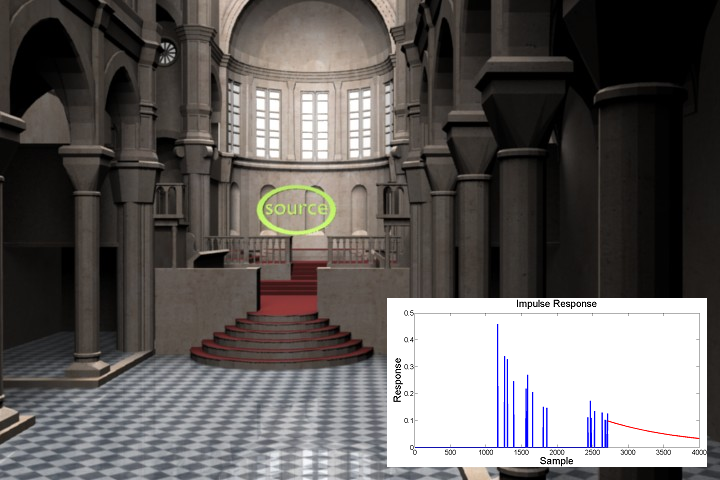# FastV: From-point Visibility Culling on Complex Models

We present an efficient technique to compute the potentially visible set (PVS) of triangles in a complex 3D scene from a viewpoint. The algorithm computes a conservative PVS at object space accuracy. Our approach traces a high number of small, volumetric frusta and computes blockers for each frustum using simple intersection tests. In practice, the algorithm can compute the PVS of CAD and scanned models composed of millions of triangles at interactive rates on a multi-core PC.We also use the visibility algorithm to accurately compute the reflection paths from a point sound source. The resulting sound propagation algorithm is 10-20X faster than prior accurate geometric acoustic methods.Fast Acoustic Simulation: We used FastV for accurate computation of reflection paths in this Cathedral model with 76.2K triangles. Our propagation algorithm performs three orders of reflections from the source (S) and compute the IR at the receiver (R) in less than 10 seconds. To the best of our knowledge, ours is the first efficient and accurate propagation algorithm to handle models of this complexity.